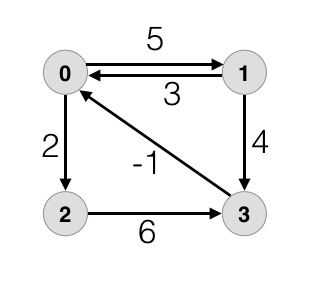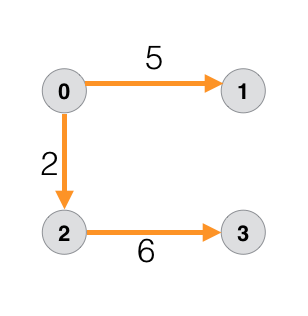# Bellman Ford Algorithm

Graph

Bellman Ford algorithm is used to find the shortest paths from a source vertex to all other vertices of a given weighted directed graph. This algorithm will work well even if the graph has a negative cycle.

### Problem

Consider the following graph with negative weight edge.In this tutorial we will be finding the shortest paths from the source vertex 0 to all other vertices.

Notations we will use are given below.
u = start vertex
v = end vertex
u --> v = directed edge from vertex u to v
w(u,v) = weight of the directed edge u --> v

Our first task is to write down the edges of the graph along with the weight.

EdgeWeight
0 --> 15
0 --> 22
1 --> 03
1 --> 34
2 --> 36
3 --> 0-1

### Point to note

• If there are N vertices then we will iterate N - 1 times to get the shortest distance.
• And we do the Nth iteration to check if there is any negative cycle.

The graph has 4 vertices so we will iterate 3 times to find shortest distance. And we will perform the 4th iteration to check if there is any negative cycle.

Note! A negative cycle in a weighted graph is a cycle whose total weight is negative.

We will need a distance array d[ ] which will hold the distance to the respective vertices from the source vertex. And a predecessor array p[ ] which will hold the predecessor of the respective vertices. Both the array will be of size N (total number of vertices).

### Steps

Step 1: We start by filling the distance array d[ ] with INFINITY and since vertex 0 is the source vertex so we will set d = 0.

Step 2: Next we will fill the predecessor array p[ ] with 0.

Step 3: We will relax all the edges of the graph by loop N-1 times.

Step 4: We will perform the Nth loop to check if the graph has any negative cycle.

Consider an edge u --> v where u is the start and v is the end vertex respectively. Relaxing an edge relax(u,v) means to find shorter path to reach v when considering edge u --> v

``````relax(u,v)
if v.d > u.d + w(u,v) then
v.d = u.d + w(u,v)
v.p = u
``````

where
v.d = distance from source vertex 0 to vertex v
u.d = distance from source vertex 0 to vertex uw(u,v) = weight of the edge u --> v
v.p = predecessor of vertex v

So, if there exists a better path to reach vertex v then, we update the distance and predecessor of vertex v.

### Why perform the Nth loop

After completing iteration (N-1) we get the shortest distance. Now in order to check if there is no negative cycle we have to perform the Nth iteration.

If there is no change in the value of distance array d[ ] in the Nth loop then the graph has no negative cycle which means we can find the shortest path. Otherwise the graph has negative cycle and we cannot find the shortest path as

### Solution

After performing the calculation we will get the following distance array d[ ].

 0 1 2 3 d 0 5 2 8

The value in the distance array d[ ] conveys the following information.
Distance from source vertex 0 to vertex 1 = 5
From vertex 0 to vertex 2 = 2
From vertex 0 to vertex 3 = 8

And the following predecessor array p[ ].

 0 1 2 3 p 0 0 0 2

The value in the predecessor array p[ ] convey the following information.
Predecessor of vertex 1 = 0
Predecessor of vertex 2 = 0
Predecessor of vertex 3 = 2

Following is the required graph.### Code in C

``````
#include <stdio.h>
#include <stdlib.h>

#define INFINITY 99999

//struct for the edges of the graph
struct Edge {
int u;  //start vertex of the edge
int v;  //end vertex of the edge
int w;  //weight of the edge (u,v)
};

//Graph - it consists of edges
struct Graph {
int V;  //total number of vertices in the graph
int E;  //total number of edges in the graph
struct Edge *edge;  //array of edges
};

void bellmanford(Graph *g, int source);
void display(int arr[], int size);

int main(void) {
//create graph
struct Graph *g = (struct Graph*)malloc(sizeof(struct Graph));
g->V = 4;  //total vertices
g->E = 6;  //total edges

//array of edges for graph
g->edge = (struct Edge*)malloc(g->E * sizeof(struct Edge));

//------- adding the edges of the graph
/*
edge(u, v)
where   u = start vertex of the edge (u,v)
v = end vertex of the edge (u,v)

w is the weight of the edge (u,v)
*/

//edge 0 --> 1
g->edge.u = 0;
g->edge.v = 1;
g->edge.w = 5;

//edge 0 --> 2
g->edge.u = 0;
g->edge.v = 2;
g->edge.w = 2;

//edge 1 --> 0
g->edge.u = 1;
g->edge.v = 0;
g->edge.w = 3;

//edge 1 --> 3
g->edge.u = 1;
g->edge.v = 3;
g->edge.w = 4;

//edge 2 --> 3
g->edge.u = 2;
g->edge.v = 3;
g->edge.w = 6;

//edge 3 --> 0
g->edge.u = 3;
g->edge.v = 0;
g->edge.w = -1;

bellmanford(g, 0);    //0 is the source vertex

return 0;
}

void bellmanford(Graph *g, int source) {
//variables
int i, j, u, v, w;

//total vertex in the graph g
int tV = g->V;

//total edge in the graph g
int tE = g->E;

//distance array
//size equal to the number of vertices of the graph g
int d[tV];

//predecessor array
//size equal to the number of vertices of the graph g
int p[tV];

//step 1: fill the distance array and predecessor array
for (i = 0; i < tV; i++) {
d[i] = INFINITY;
p[i] = 0;
}

//mark the source vertex
d[source] = 0;

//step 2: relax edges |V| - 1 times
for(i = 1; i <= tV-1; i++) {
for(j = 0; j < tE; j++) {
//get the edge data
u = g->edge[j].u;
v = g->edge[j].v;
w = g->edge[j].w;

if(d[u] != INFINITY && d[v] > d[u] + w) {
d[v] = d[u] + w;
p[v] = u;
}
}
}

//step 3: detect negative cycle
//if value changes then we have a negative cycle in the graph
//and we cannot find the shortest distances
for(i = 0; i < tE; i++) {
u = g->edge[i].u;
v = g->edge[i].v;
w = g->edge[i].w;
if(d[u] != INFINITY && d[v] > d[u] + w) {
printf("Negative weight cycle detected!\n");
return;
}
}

//No negative weight cycle found!
//print the distance and predecessor array
printf("Distance array: ");
display(d, tV);
printf("Predecessor array: ");
display(p, tV);
}

void display(int arr[], int size) {
int i;
for(i = 0; i < size; i ++) {
printf("%d ", arr[i]);
}
printf("\n");
}
``````

### Time complexity

Bellman Ford algorithm has a time complexity of O(VE) where V is the total number of vertices in the graph and E is the total number of edges in the graph.